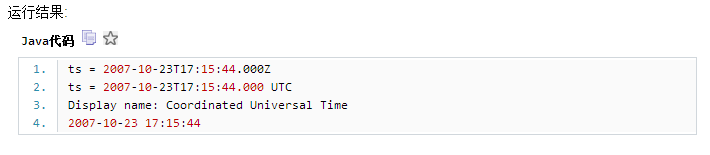JAVA 怎么将时间戳转为2015-10-05T21:02:49.228Z 这种格式

7个回答

String time = DateUtil.format(new Date(),"yyyy-MM-ddTHH:mm:ss.SSSZ");

SimpleDateFormat sdf= new SimpleDateFormat("yyyy-dd-MM HH:mm:ss");
sdf.prase(str)

``````//change formation of long to Date
private String transformDataFormation(long source){
SimpleDateFormat sdf= new SimpleDateFormat("yyyy-dd-MM  HH:mm:ss");
//前面的lSysTime是秒数，先乘1000得到毫秒数，再转为java.util.Date类型
java.util.Date dt = new Date(source);
String sDateTime = sdf.format(dt);  //得到精确到秒的表示：08/31/2006 21:08:00
Log.i("blb", "---------time:" + sDateTime);
return sDateTime;
}
``````

``````String time =  DateUtil.format(new Date(),"yyyy-MM-dd");

``````3 年多之前 回复
`````` public static Date fromISODate(String time){
if(!time.matches("\\d{4}-\\d{2}-\\d{2}T\\d{2}:\\d{2}:\\d{2}.\\d{3}Z")){
return null;
}
time=time.replaceFirst("T", " ").replaceFirst(".\\d{3}Z", "");
Date date=formatDate(time);
Calendar ca=Calendar.getInstance();
ca.setTime(date);
return ca.getTime();
}
``````

http://www.w3chtml.com/schema/xml-schema-date-time.html

# **Java转换UTC时间:2015-10-13T14:15:44.000Z **

public class TimeZoneTest

{

public static void main (String args[])

{

try

{

String ts = "2007-10-23T17:15:44.000Z";

System.out.println("ts = " + ts);

ts = ts.replace("Z", " UTC");

System.out.println("ts = " + ts);

SimpleDateFormat sdf = new SimpleDateFormat("yyyy-MM-dd'T'HH:mm:ss.SSS Z");

``````                     Date dt = sdf.parse(ts);

TimeZone tz = sdf.getTimeZone();
Calendar c = sdf.getCalendar();
System.out.println("Display name: " +
``````

tz.getDisplayName());

System.out.println(getString(c));

}

catch(ParseException pe)

{

System.out.println("Error offset: " + pe.getErrorOffset());

pe.printStackTrace();

}

}

``````    private static String getString(Calendar c)
{
StringBuffer result = new StringBuffer();
result.append(c.get(Calendar.YEAR));
result.append("-");
result.append((c.get(Calendar.MONTH) + 1));
result.append("-");
result.append(c.get(Calendar.DAY_OF_MONTH));
result.append(" ");
result.append(c.get(Calendar.HOUR_OF_DAY));
result.append(":");
result.append(c.get(Calendar.MINUTE));
result.append(":");
result.append(c.get(Calendar.SECOND));
return result.toString();
}
``````

}